Courses

# First Law of Thermodynamics (Part - 1) - Physics, Solution by DC Pandey NEET Notes | EduRev

## JEE : First Law of Thermodynamics (Part - 1) - Physics, Solution by DC Pandey NEET Notes | EduRev

The document First Law of Thermodynamics (Part - 1) - Physics, Solution by DC Pandey NEET Notes | EduRev is a part of the JEE Course DC Pandey Solutions for NEET Physics.
All you need of JEE at this link: JEE

Introductory Exercise 18.1

Ques 1: A gas in a cylinder is held at a constant pressure of 1.7 × 105 Pa and is cooled and compressed from 1.20 m3 to 0.8 m3. The internal energy of the gas decreases by 1.1 × 105 J.
(a) Find the work done by the gas.
(b) Find the magnitude of the heat flow into or out of the gas, and state the direction o f heat flow.
(c) Does it matter whether or not the gas is ideal ?
Sol:
(a) Work done by gas under constant pressure is
W = P(Vf + Vi)
= (1.7 × 105)(0.8 - 1.2)

= -6.8 × 104J
(b) Q = W + ΔU
= -6.8 × 104 -1.1 × 105
or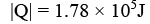As Q is negative. So, net heat flow is out of the gas.

Ques 2: A thermodynamic system undergoes a cyclic process as shown in figure.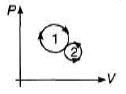(a) over one complete cycle, does the system do positive or negative work.
(b) over one complete cycle, does heat flow into or out of the system.
(c) In each of the loops 1 and 2, does heat flow into or out of the system.
Sol:
(a) W1 = + ve, as cycle is clockwise
W2 = - ve as cycle is anticlockwise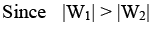∴ Net work done by the system is positive.
(b) In cyclic process, Qnet = Wnet
Since Wnet is positive.
So, Qnet is also positive. So, heat flows into the system.
(c) W1 = Q= + ve, so, into the system.
W2 = Q2 = -ve, so, of the system.

Ques 3: A well insulated box contains a partition dividing the box into two equal volumes as shown in figure. Initially the left hand side contains an ideal monoatomic gas and the other half is a vacuum. The partition is suddenly removed so that the gas now is contained throughout the entire box.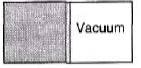(a) Does the temperature of the gas change?
(b) Does the internal energy of the system change?
(c) Does the gas work?
Sol:
Q = 0 as the box is insulated.
Wgas = 0 as on the right hand side there is vacuum.
ΔU = Q - W = 0
ΔT is also zero, as U ∝ T

Ques 4: How many moles of helium at temperature 300 K and 1.00 atm pressure are needed to make the internal energy of the gas 100 J?
Sol:
Helium is monoatomic. So, degree of freedom f = 3.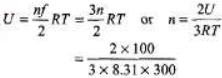= 2.67 × 10-2 mol

Ques 5: A 1.0 kg bar of copper is heated at atmospheric pressure (1.01 × 105 N/m2). If its temperature increases from 20°C to 50°C, calculate the change in its internal energy. α = 7.0 × 10-6 per°C, ρ = 8.92 × 103 kg/m3 and c =387 J/kg-°C
Sol: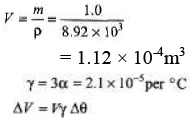= (1.12 × 10-4)(2.1 × 10-5)(30) = 7.056 × 10-8m3
W = PΔV
= (1.01 × 105)(7.056 × 10-8) = 7.13 × 10-3J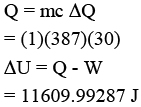Offer running on EduRev: Apply code STAYHOME200 to get INR 200 off on our premium plan EduRev Infinity!

132 docs

,

,

,

,

,

,

,

,

,

,

,

,

,

,

,

,

,

,

,

,

,

,

,

,

;